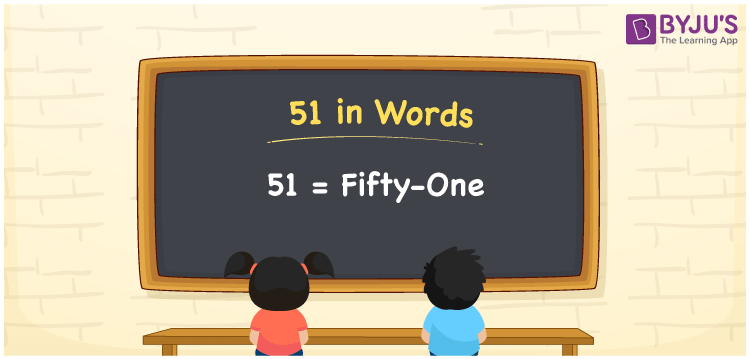# 51 in Words

51 in words is written as “Fifty-one”. 51 is a two-digit number that has two place values, ones and tens. It represents a count, quantity or value. To represent 51 in words, we should know how to read and write it in English since it is the most commonly used language. Also, learning to spell numbers in words is necessary to utilise in real-life situations. For example, Samrah saved Fifty-one Rupees out of shopping today. Learn how to write 51 in words in this article.

 51 in words Fifty-one Fifty-one in Numbers 51

## 51 in English Words

We generally write numbers in words using the English alphabet. Thus, we can read 51 in English as “Fifty-one”.## How to Write 51 in Words?

To convert 51 in words, we need to check the place value of its digits. Since 51 is a two-digit number, thus, place value of 5 is tens and 1 is ones. The order of place values of digits in a number is in accordance with the Indian numbering system, such as:

• Ones
• Tens
• Hundreds
• Thousands
• Ten-thousands
• Hundred-thousands or Lakhs

This can be done as follows.

 Tens Ones 5 1

This can be written as:

5 × Ten + 1 × One

= 5 × 10 + 1 × 1

= 50 + 1

= 51

= Fifty-one

Thus, 51 in words is written as Fifty-one.

51 is a natural number that precedes 52 and succeeds 50.

51 in words – Fifty-one

Is 51 an odd number? – Yes

Is 51 an even number? – No

Is 51 a perfect square number? – No

Is 51 a perfect cube number? – No

Is 51 a prime number? – No

Is 51 a composite number? – Yes

## Frequently Asked Questions on 51 in Words

Q1

### How do you write 51 in English?

We can write 51 in English words as “Fifty-one”.
Q2

### What is 351 in English?

351 is written as Three hundred and Fifty-one in English.
Q3

### Is 51 an even number or an odd number?

51 is an odd number since it is not completely divisible by 2.